Next: Locally linear prediction Up: Nonlinear prediction Previous: Simple nonlinear prediction

## Finding unstable periodic orbits

As an application of simple nonlinear phase space prediction, let us discuss a method to locate unstable periodic orbits embedded in a chaotic attractor. This is not the place to review the existing methods to solve this problem, some references include [47, 48, 49, 50]. The TISEAN package contains a routine that implements the requirement that for a period p orbit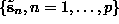of a dynamical system like Eq.() acting on delay vectors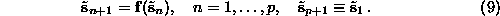With unit delay, the p delay vectors contain p different scalar entries, and Eq.() defines a root of a system of p nonlinear equations in p dimensions. Multidimensional root finding is not a simple problem. The standard Newton method has to be augmented by special tricks in order to converge globally. Some such tricks, in particular means to select different solutions of Eq.() are implemented in . Similarly to the problems encountered in nonlinear noise reduction, solving Eq.() exactly is particularly problematic since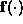is unknown and must be estimated from the data. In Ref. , approximate solutions are found by performing just one iteration of the Newton method for each available time series point. We prefer to look for a least squares solution by minimizing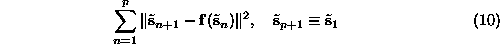instead. The routine upo uses a standard Levenberg-Marquardt algorithm to minimize (). For this it is necessary thatis smooth. Therefore we cannot use the simple nonlinear predictor based on locally constant approximations and we have to use a smooth kernel version, Eq.(), instead. With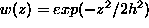, the kernel bandwidth h determines the degree of smoothness of. Trying to start the minimization with all available time series segments will produce a number of false minima, depending on the value of h. These have to be distinguished from the true solutions by inspection. On the other hand, we can reach solutions of Eq.() which are not closely visited in the time series at all, an important advantage over close return methods .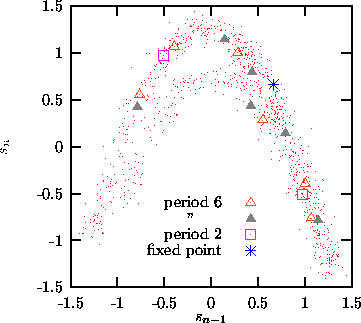Figure:   Orbits of period six, or a sub-period thereof, of the Hénon map, determined from noisy data. The Hénon attractor does not have a period three orbit.

It should be noted that, depending on h, we may always find good minima of (), even if no solution of Eq.(), or not even a truly deterministic dynamics exists. Thus the finding of unstable periodic orbits in itself is not a strong indicator of determinism. We may however use the cycle locations or stabilities as a discriminating statistics in a test for nonlinearity, see Sec.. While the orbits themselves are found quite easily, it is surprisingly difficult to obtain reliable estimates of their stability in the presence of noise. In upo, a small perturbation is iterated along the orbit and the unstable eigenvalue is determined by the rate of its separation from the periodic orbit.

The user of upo has to specify the embedding dimension, the period (which may also be smaller) and the kernel bandwidth. For efficiency, one may choose to skip trials with very similar points. Orbits are counted as distinct only when they differ by a specified amount. The routine finds the orbits, their expanding eigenvalue, and possible sub-periods. Figureshows the determination of all period six orbits from 1000 iterates of the Hénon map, contaminated by 10% Gaussian white noise.Next: Locally linear prediction Up: Nonlinear prediction Previous: Simple nonlinear prediction

Thomas Schreiber
Wed Jan 6 15:38:27 CET 1999